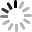×

×

# php实现字节Byte转换为KB、MB、GB、TB换算转换方法

### 详情内容

byte 字节

byte就是字节

1byte=8bit

1byte就是1B

KB(千字节)

kB(Kilobyte)，是一种资讯计量单位，是计算机数据存贮器存储单位字节的多倍形式。现今通常在标识内存等具有一般容量的储存媒介之储存容量时使用。

B 1B= 8b

KB 1KB=1024B

MB 1MB=1024KB

GB 1GB=1024MB

TB 1TB=1024GB

PHP实现字节数Byte转换为KB、MB、GB、TB的方法

```function get_filesize(\$num){
\$p = 0;
\$format='bytes';
if(\$num>0 && \$num<1024){
\$p = 0;
return number_format(\$num).' '.\$format;
}
if(\$num>=1024 && \$num<pow(1024, 2)){
\$p = 1;
\$format = 'KB';
}
if (\$num>=pow(1024, 2) && \$num<pow(1024, 3)) {
\$p = 2;
\$format = 'MB';
}
if (\$num>=pow(1024, 3) && \$num<pow(1024, 4)) {
\$p = 3;
\$format = 'GB';
}
if (\$num>=pow(1024, 4) && \$num<pow(1024, 5)) {
\$p = 3;
\$format = 'TB';
}
\$num /= pow(1024, \$p);
return number_format(\$num, 1).' '.\$format;
}```

Byte转换为KB、MB、GB、TB显示效果图：×
••×

×

### 选择支付方式

••